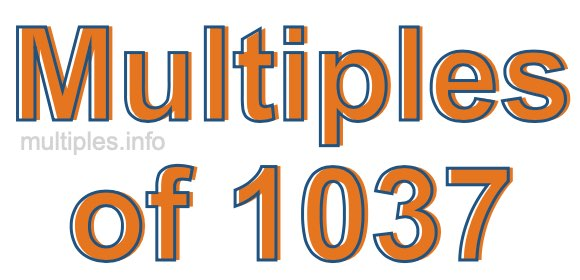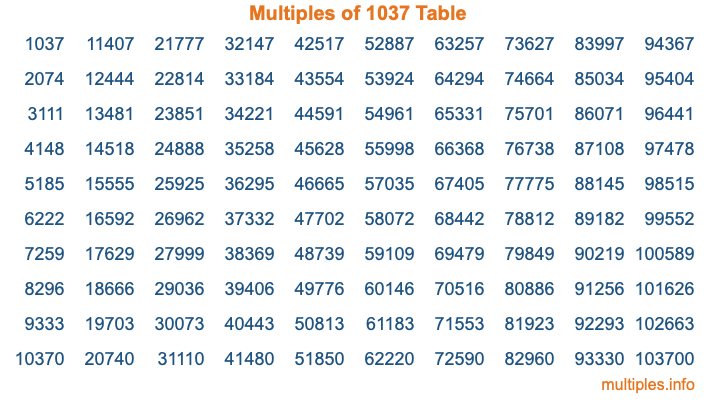Multiples of 1037Welcome to the Multiples of 1037 page. Here we will first teach you everything you will ever need to know about the multiples of 1037, and then give you a study guide summary of everything we taught you to make sure you remember it all. Use this page to look up facts and learn information about the multiples of 1037. This page will make you a multiples of one thousand thirty-seven expert!

Definition of Multiples of 1037
Multiples of 1037 are all the numbers that when divided by 1037 equal an integer. Each of the multiples of 1037 are called a multiple. A multiple of 1037 is created by multiplying 1037 by an integer.

Therefore, to create a list of multiples of 1037, you start with 1 multiplied by 1037, then 2 multiplied by 1037, then 3 multiplied by 1037, and so on for as long as you want. Thus, the list of the first five multiples of 1037 is 1037, 2074, 3111, 4148, and 5185. To see a larger list of multiples of 1037, see the printable image of Multiples of 1037 further down on this page. We also have a category where you can choose any nth multiple of 1037.

Multiples of 1037 Checker
The Multiples of 1037 Checker below checks to see if any number of your choice is a multiple of 1037. In other words, it checks to see if there is any number (integer) that when multiplied by 1037 will equal your number. To do that, we divide your number by 1037. If the the quotient is an integer, then your number is a multiple of 1037.

Is  a multiple of 1037?

Least Common Multiple of 1037 and ...
A Least Common Multiple (LCM) is the lowest multiple that two or more numbers have in common. This is also called the smallest common multiple or lowest common multiple and is useful to know when you are adding our subtracting fractions. Enter one or more numbers below (1037 is already entered) to find the LCM.

Check out our LCM Calculator if you need more details about the Least Common Multiple or if you need the LCM for different numbers for adding and subtraction fractions.

nth Multiple of 1037
As we stated above, 1037 is the first multiple of 1037, 2074 is the second multiple of 1037, 3111 is the third multiple of 1037, and so on. Enter a number below to find the nth multiple of 1037.

th multiple of 1037

Multiples of 1037 vs Factors of 1037
1037 is a multiple of 1037 and a factor of 1037, but that is where the similarities end. All postive multiples of 1037 are 1037 or greater than 1037. All positive factors of 1037 are 1037 or less than 1037.

Below is the beginning list of multiples of 1037 and the factors of 1037 so you can compare:

Multiples of 1037: 1037, 2074, 3111, 4148, 5185, etc.

Factors of 1037: 1, 17, 61, 1037

As you can see, the multiples of 1037 are all the numbers that you can divide by 1037 to get a whole number. The factors of 1037, on the other hand, are all the whole numbers that you can multiply by another whole number to get 1037.

It's also interesting to note that if a number (x) is a factor of 1037, then 1037 will also be a multiple of that number (x).

Multiples of 1037 vs Divisors of 1037
The divisors of 1037 are all the integers that 1037 can be divided by evenly. Below is a list of the divisors of 1037.

Divisors of 1037: 1, 17, 61, 1037

The interesting thing to note here is that if you take any multiple of 1037 and divide it by a divisor of 1037, you will see that the quotient is an integer.

Multiples of 1037 Table
Below is an image of the first 100 multiples of 1037 in a table. The table is in chronological order, column by column. The first column has the first ten multiples of 1037, the second column has the next ten multiples of 1037, and so on.The Multiples of 1037 Table is also referred to as the 1037 Times Table or Times Table of 1037. You are welcome to print out our table for your studies.

Negative Multiples of 1037
Although not often discussed or needed in math, it is worth mentioning that you can make a list of negative multiples of 1037 by multiplying 1037 by -1, then by -2, then by -3, and so on, to get the following list of negative multiples of 1037:

-1037, -2074, -3111, -4148, -5185, etc.

Multiples of 1037 Summary
Below is a summary of important Multiples of 1037 facts that we have discussed on this page. To retain the knowledge on this page, we recommend that you read through the summary and explain to yourself or a study partner why they hold true.

There are an infinite number of multiples of 1037.

A multiple of 1037 divided by 1037 will equal a whole number.

1037 divided by a factor of 1037 equals a divisor of 1037.

The nth multiple of 1037 is n times 1037.

The largest factor of 1037 is equal to the first positive multiple of 1037.

1037 is a multiple of every factor of 1037.

1037 is a multiple of 1037.

A multiple of 1037 divided by a divisor of 1037 equals an integer.

1037 divided by a divisor of 1037 equals a factor of 1037.

Any integer times 1037 will equal a multiple of 1037.

Multiples of a Number
Here you can get the multiples of another number, all with the same attention to detail as we did for multiples of 1037 on this page.

Multiples of
Multiples of 1038
Did you find our page about multiples of one thousand thirty-seven educational? Do you want more knowledge? Check out the multiples of the next number on our list!